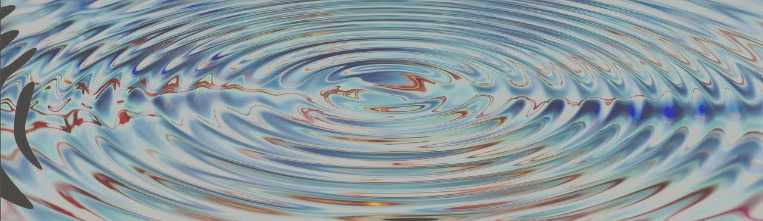top of pagePiezo Accelerometer Tutorial

What is Vibration?

# Basics of Vibration 1

## Vibration

A body is said to vibrate when the body as a whole or parts of it are oscillating about a rest position. (For the moment we are looking at oscillations that are cyclic or periodic.)

The number of times a complete motion cycle takes place during one second is called frequency.

The dimension of the frequency is called Hertz [Hz]

1 Hz equals to 1/sec (or 1 cycle per second)

If the oscillating motion consists of a single component occurring at a single frequency, like for example a tuning fork, the motion is called harmonic or sinusoidal.

The vibration of a real machine is normally much more complex and consists of many components with different frequencies occurring simultaneously.© by Wikipedia, the free encyclopedia

## Three different Ways to describe Sinusoidal Vibration

In the following we will explore the sinusoidal vibration in more details. There are three different ways to describe and quantify the vibration:

Displacement, velocity and acceleration

### Displacement

The curve we obtain over the time line is called a sine or sinus whereby

D = amplitude
= largest excursion positive or negative

T = period
= time to complete one complete cycle

= frequency   f = 1/T
= number of cycles per secondDisplacement

### Velocity

The velocity is another mean to describe vibration.

The velocity is also a sine function but it is leading the displacement by a certain phase shift.

The phase shift is 1/4 of a period.

We say also the phase angle is = 90°

(the complete period is referred to 360°)

V = velocity amplitude
Frequency f = 1 / TNote: The scale of the velocity amplitude has been chosen in a way that it appears equal to the displacement amplitude

Velocity

### Acceleration

A third mean finally to describe vibration is the acceleration

The acceleration is sinusoidal like the displacement however it points exactly in the opposite direction.

We can also say the acceleration is leading the displacement half a cycle or the phase shift is 180°

A = acceleration amplitude

Frequency f = 1 / TNote: The scale of the acceleration amplitude has been chosen in a way that it appears equal to the displacement amplitude

Acceleration
bottom of page